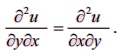Home | | Business Maths 11th std | Successive partial derivatives

# Successive partial derivatives

Consider the functionu u = f( x, y) .

Successive partial derivatives

Consider the functionu u = f( x, y) . From this we can findare functions of x and y, then they may be differentiated partially again with respect to either of the independent variables, (x or y) denoted by.

These derivatives are called second order partial derivatives. Similarly, we can find the third order partial derivatives, fourth order partial derivatives etc., if they exist. The process of finding such partial derivatives are called successive partial derivatives.

If we differentiate u = f(x,y) partially with respect to x and again differentiating partially with respect to y, we obtain.

Similarly, if we differentiate u = f(x,y)  partially with respect to y and again differentiating partially with respect to xNOTE

If u(x,y)is a continuous function of x and y then## Homogeneous functions

A function f(x,y) of two independent variables x and y is said to be homogeneous in x and y of degree n if f (tx , ty ) = t n f ( x, y) for t > 0.

Tags : Applications of Differentiation , 11th Business Mathematics and Statistics(EMS) : Chapter 6 : Applications of Differentiation
Study Material, Lecturing Notes, Assignment, Reference, Wiki description explanation, brief detail
11th Business Mathematics and Statistics(EMS) : Chapter 6 : Applications of Differentiation : Successive partial derivatives | Applications of Differentiation